# Test: Electrical Machines- 6

## 10 Questions MCQ Test RRB JE for Electrical Engineering | Test: Electrical Machines- 6

Description
Attempt Test: Electrical Machines- 6 | 10 questions in 30 minutes | Mock test for Electrical Engineering (EE) preparation | Free important questions MCQ to study RRB JE for Electrical Engineering for Electrical Engineering (EE) Exam | Download free PDF with solutions
QUESTION: 1

### A 2 pole, 50 Hz single phase induction motor running at 2600 rpm has an effective rotor resistance and reactance each of 0.5 Ω. The stator impedance and magnetizing current are to be neglected. The frequencies of rotor current in forward branch and in backward branch are to be respectively

Solution:

Synchronous speed,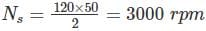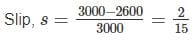Frequency of forward component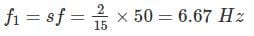Frequency of backward component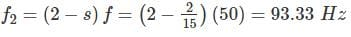*Answer can only contain numeric values
QUESTION: 2

### A 250 V, 700 W, 50 Hz, 6 pole single phase induction motor is rotating is clockwise direction with a speed of 960 rpm. The rotor resistance at standstill is 10 Ω. What is the effective rotor resistance is the backward branch of the equivalent circuit in ohms?

Solution:

Synchronous speed, NS = 120f/p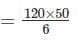= 1000 rpm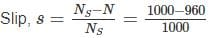= 0.04
Resistance of backward field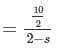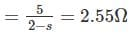QUESTION: 3

### The direction of rotation of a single-phase capacitor run induction motor is reversed by

Solution:

By interchanging the terminals of the auxiliary winding, the direction of rotation of the rotating magnetic field get reverse and hence rotor.

QUESTION: 4

A 50 Hz, 6 pole, single phase induction motor is rotating in the clockwise direction at a speed of 940 rpm. The slip of motor in the direction of rotation and opposite direction of motor will be respectively.

Solution: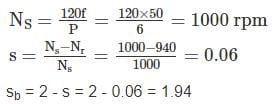QUESTION: 5

The starting current and torque of a three phase induction motor on direct line starting is 45 A and 600 Nm respectively. What are the corresponding values with star delta starter.

Solution:

Starting current with star delta starter
1/3 of direct on line starting current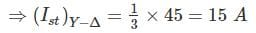Starting torque (Tst) with Y - ∆ starter = 1/3 of DOL starting torque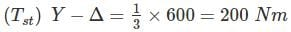*Answer can only contain numeric values
QUESTION: 6

The value of the starting capacitor required to produce 90° phase difference between the currents in the main and auxiliary windings will be – (in μF)

Solution: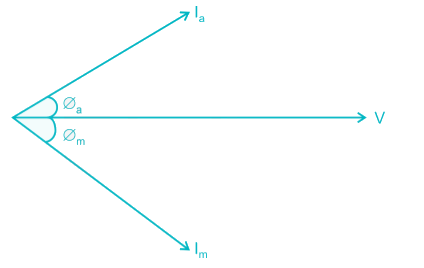Given that ∅a + ∅m = 90°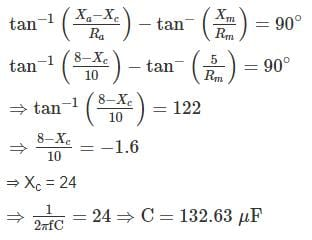*Answer can only contain numeric values
QUESTION: 7

An 8 pole 40 kW, 125 V, 50 Hz single phase motor is running at speed at 720 rpm with forward field torque of 200 N-m end backward field torque of 40 N-m. If friction and winding losses are 20 w, what is the mechanical power output in watts?

Solution:

Synchronous watts = Net torque
= Forward torque – Backward torque
= 200 – 40
= 160 N-m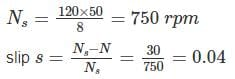watts converted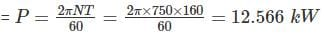Watts converted = 12.566 (1 - s)
= 12.566 (1 – 0.04)
= 12063.3 W
Mechanical power output = 12063.3 – 20
= 12043.3 W

QUESTION: 8

A 6 pole, 350 W, 110 V, 50 Hz single phase motor is running at 960 rpm with forward field torque of 220 N-m and backward field torque of 30 N-m. If friction and winding losses are 10 W, What is the mechanical power output?

Solution:

Synchronous watt = net torque
= Forward torque – Backward torque
= 220 – 30
= 190 N – m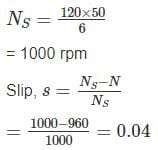Watts converted = 190 (1 – 0.04)
= 182.4 W
Mechanical power output = 182.4 – 10
= 172.4 W

QUESTION: 9

Determine the current drawn from the main as a fraction of full load current of an auto transformer starter for an induction motor required to start the motor with 50 percent of full-load torque. The short circuit current of the motor is 6 times the full load current and full load slip is 0.05.

Solution:

We know that,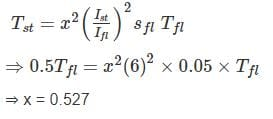Current drawn from the supply =  x2 Ist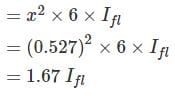*Answer can only contain numeric values
QUESTION: 10

A induction motor is started in first case by autotransformer with 3/4th tapping and is second case by a star delta starter. What is the ratio of supply current at starting of first case to second one upto two decimal places

Solution:

In the case of autotransformer starter, per phase starting current, Ist = x2Isc
= (0.75)2Isc
= 0.5625 Isc
For star delta starter,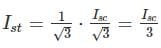= 0.33 Isc
The ratio of first case to second is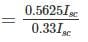= 1.6876
≈ 1.69Use Code STAYHOME200 and get INR 200 additional OFF Use Coupon Code